# OpenCV-Python图像的几何变换

• 你将会学到将不同的几何变换应用于图像，如平移、旋转、仿射变换等。
• 你会学到如下函数：cv.getPerspectiveTransform

## OpenCV变换

OpenCV 提供了两个变换函数， cv.warpAffinecv.getPerspectiveTransform ，用这两个函数就可以完成所有类型的变换。 cv.warpAffine 输入为 2×3 的变换矩阵， cv.getPerspectiveTransform 输入为 2×3 的变换矩阵。

## OpenCV缩放

import numpy as np
import cv2 as cv
res = cv.resize(img,None,fx=2, fy=2, interpolation = cv.INTER_CUBIC)
#OR
height, width = img.shape[:2]
res = cv.resize(img,(2*width, 2*height), interpolation = cv.INTER_CUBIC)


## OpenCV平移变换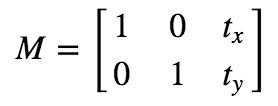import numpy as np
import cv2 as cv
rows,cols = img.shape
M = np.float32([[1,0,100],[0,1,50]])
dst = cv.warpAffine(img,M,(cols,rows))
cv.imshow('img',dst)
cv.waitKey(0)
cv.destroyAllWindows()


### 警告

cv.warpAffine 函数的第三个参数是输出图像的大小，其形式应为（宽度、高度）。记住宽度=列数，高度=行数。## OpenCV旋转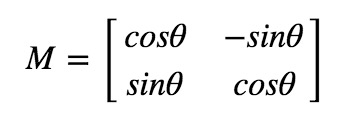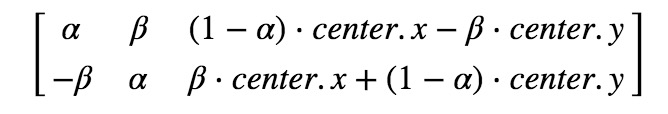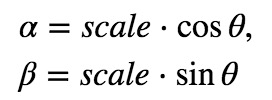img = cv.imread('messi5.jpg',0)
rows,cols = img.shape
# cols-1 and rows-1 are the coordinate limits.
M = cv.getRotationMatrix2D(((cols-1)/2.0,(rows-1)/2.0),90,1)
dst = cv.warpAffine(img,M,(cols,rows))## OpenCV仿射变换

img = cv.imread('drawing.png')
rows,cols,ch = img.shape
pts1 = np.float32([[50,50],[200,50],[50,200]])
pts2 = np.float32([[10,100],[200,50],[100,250]])
M = cv.getAffineTransform(pts1,pts2)
dst = cv.warpAffine(img,M,(cols,rows))
plt.subplot(121),plt.imshow(img),plt.title('Input')
plt.subplot(122),plt.imshow(dst),plt.title('Output')
plt.show()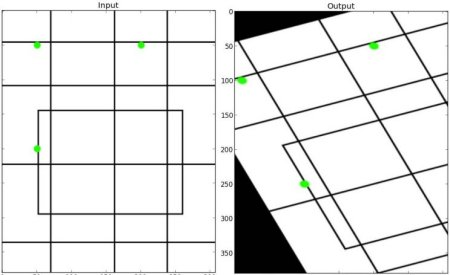## OpenCV透视变换

img = cv.imread('sudoku.png')
rows,cols,ch = img.shape
pts1 = np.float32([[56,65],[368,52],[28,387],[389,390]])
pts2 = np.float32([[0,0],[300,0],[0,300],[300,300]])
M = cv.getPerspectiveTransform(pts1,pts2)
dst = cv.warpPerspective(img,M,(300,300))
plt.subplot(121),plt.imshow(img),plt.title('Input')
plt.subplot(122),plt.imshow(dst),plt.title('Output')
plt.show()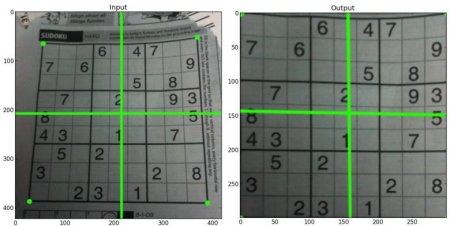• 回顶